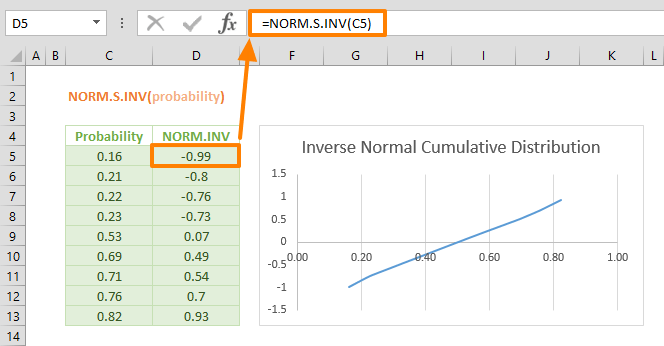The Excel NORM.S.INV function is a Statistical formula that calculates and returns the inverse of the standard normal cumulative distribution with a given probability. Frequently used in statistics and data analysis, the standard normal distribution is a normal distribution with a mean of zero (0), and a standard deviation of one (1). In this guide, we’re going to show you how to use the NORM.S.INV (NORM S INV) function and also go over some tips and error handling methods.

## Supported versions

• Excel 2010 and later (In earlier versions of Excel, only the NORMSINV function is supported.)

## NORM.S.INV Function Syntax

NORM.S.INV(probability)

## Arguments

 probability The probability corresponding to the standard normal distribution.

## Examples

### Probability density function

The NORM.S.INV function calculates the value which proves the standard cumulative normal distribution function, based on the specified mean and standard deviation values. Essentially, Excel calculates and returns the probability using the formula: NORM.S.DIST(z, TRUE) = probability.

=NORM.S.INV(0.11,26.44,11.17)=NORM.S.INV(NORM.S.DIST(0.69,TRUE)) returns 0.69## Tips for Using the NORM.S.INV Function

• You can use the STDEV.P or STDEV.S functions to calculate the standard deviation for the entire population, or a sample, respectively.
• You can use the AVERAGE function to calculate the mean of a data set.
• The NORMSINV function is the older version of the NORM.S.INV function. We recommend not using the NORMSINV function, unless you are using an Excel version that is older than 2010.
• Below is a list of other similar functions:
• NORM.DIST function returns the normal distribution.
• NORM.S.DIST function returns the standard normal distribution.
• NORM.INV function returns the inverse of the cumulative normal distribution.

## Issues

• The S.INV function returns #NUM! error value;
• When the standard_dev argument is ≤ 0
• If the probability ≤ 0 or if the probability ≥ 1